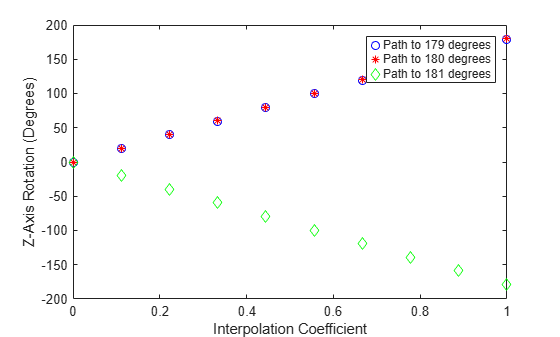# slerp

Spherical linear interpolation

## Syntax

``q0 = slerp(q1,q2,T)``

## Description

example

````q0 = slerp(q1,q2,T)` spherically interpolates between `q1` and `q2` by the interpolation coefficient `T`. The function always chooses the shorter interpolation path between `q1` and `q2`.```

## Examples

collapse all

Create two quaternions with the following interpretation:

1. `a` = 45 degree rotation around the z-axis

2. `c` = -45 degree rotation around the z-axis

```a = quaternion([45,0,0],'eulerd','ZYX','frame'); c = quaternion([-45,0,0],'eulerd','ZYX','frame');```

Call `slerp` with the quaternions `a` and `c` and specify an interpolation coefficient of 0.5.

```interpolationCoefficient = 0.5; b = slerp(a,c,interpolationCoefficient);```

The output of `slerp`, `b`, represents an average rotation of `a` and `c`. To verify, convert `b` to Euler angles in degrees.

`averageRotation = eulerd(b,'ZYX','frame')`
```averageRotation = 1×3 0 0 0 ```

The interpolation coefficient is specified as a normalized value between `0` and `1`, inclusive. An interpolation coefficient of `0` corresponds to the `a` quaternion, and an interpolation coefficient of `1` corresponds to the `c` quaternion. Call `slerp` with coefficients `0` and `1` to confirm.

```b = slerp(a,c,[0,1]); eulerd(b,'ZYX','frame')```
```ans = 2×3 45.0000 0 0 -45.0000 0 0 ```

You can create smooth paths between quaternions by specifying arrays of equally spaced interpolation coefficients.

```path = 0:0.1:1; interpolatedQuaternions = slerp(a,c,path);```

For quaternions that represent rotation only about a single axis, specifying interpolation coefficients as equally spaced results in quaternions equally spaced in Euler angles. Convert `interpolatedQuaternions` to Euler angles and verify that the difference between the angles in the path is constant.

```k = eulerd(interpolatedQuaternions,'ZYX','frame'); abc = abs(diff(k))```
```abc = 10×3 9.0000 0 0 9.0000 0 0 9.0000 0 0 9.0000 0 0 9.0000 0 0 9.0000 0 0 9.0000 0 0 9.0000 0 0 9.0000 0 0 9.0000 0 0 ```

Alternatively, you can use the `dist` function to verify that the distance between the interpolated quaternions is consistent. The `dist` function returns angular distance in radians; convert to degrees for easy comparison.

`def = rad2deg(dist(interpolatedQuaternions(2:end),interpolatedQuaternions(1:end-1)))`
```def = 1×10 9.0000 9.0000 9.0000 9.0000 9.0000 9.0000 9.0000 9.0000 9.0000 9.0000 ```

The SLERP algorithm interpolates along a great circle path connecting two quaternions. This example shows how the SLERP algorithm minimizes the great circle path.

Define three quaternions:

1. `q0` - quaternion indicating no rotation from the global frame

2. `q179` - quaternion indicating a 179 degree rotation about the z-axis

3. `q180` - quaternion indicating a 180 degree rotation about the z-axis

4. `q181` - quaternion indicating a 181 degree rotation about the z-axis

```q0 = ones(1,'quaternion'); q179 = quaternion([179,0,0],'eulerd','ZYX','frame'); q180 = quaternion([180,0,0],'eulerd','ZYX','frame'); q181 = quaternion([181,0,0],'eulerd','ZYX','frame');```

Use `slerp` to interpolate between `q0` and the three quaternion rotations. Specify that the paths are traveled in 10 steps.

```T = linspace(0,1,10); q179path = slerp(q0,q179,T); q180path = slerp(q0,q180,T); q181path = slerp(q0,q181,T);```

Plot each path in terms of Euler angles in degrees.

```q179pathEuler = eulerd(q179path,'ZYX','frame'); q180pathEuler = eulerd(q180path,'ZYX','frame'); q181pathEuler = eulerd(q181path,'ZYX','frame'); plot(T,q179pathEuler(:,1),'bo', ... T,q180pathEuler(:,1),'r*', ... T,q181pathEuler(:,1),'gd'); legend('Path to 179 degrees', ... 'Path to 180 degrees', ... 'Path to 181 degrees') xlabel('Interpolation Coefficient') ylabel('Z-Axis Rotation (Degrees)')```The path between `q0` and `q179` is clockwise to minimize the great circle distance. The path between `q0` and `q181` is counterclockwise to minimize the great circle distance. The path between `q0` and `q180` can be either clockwise or counterclockwise, depending on numerical rounding.

Create two quaternions.

```q1 = quaternion([75,-20,-10],'eulerd','ZYX','frame'); q2 = quaternion([-45,20,30],'eulerd','ZYX','frame');```

Define the interpolation coefficient.

`T = 0:0.01:1;`

Obtain the interpolated quaternions.

`quats = slerp(q1,q2,T);`

Obtain the corresponding rotate points.

`pts = rotatepoint(quats,[1 0 0]);`

Show the interpolated quaternions on a unit sphere.

```figure [X,Y,Z] = sphere; surf(X,Y,Z,'FaceColor',[0.57 0.57 0.57]) hold on; scatter3(pts(:,1),pts(:,2),pts(:,3)) view([69.23 36.60]) axis equal```Note that the interpolated quaternions follow the shorter path from `q1` to `q2`.

## Input Arguments

collapse all

Quaternion to interpolate, specified as a scalar, vector, matrix, or multidimensional array of quaternions.

`q1`, `q2`, and `T` must have compatible sizes. In the simplest cases, they can be the same size or any one can be a scalar. Two inputs have compatible sizes if, for every dimension, the dimension sizes of the inputs are either the same or one of them is 1.

Data Types: `quaternion`

Quaternion to interpolate, specified as a scalar, vector, matrix, or multidimensional array of quaternions.

`q1`, `q2`, and `T` must have compatible sizes. In the simplest cases, they can be the same size or any one can be a scalar. Two inputs have compatible sizes if, for every dimension, the dimension sizes of the inputs are either the same or one of the dimension sizes is 1.

Data Types: `quaternion`

Interpolation coefficient, specified as a scalar, vector, matrix, or multidimensional array of numbers with each element in the range [0,1].

`q1`, `q2`, and `T` must have compatible sizes. In the simplest cases, they can be the same size or any one can be a scalar. Two inputs have compatible sizes if, for every dimension, the dimension sizes of the inputs are either the same or one of the dimension sizes is 1.

Data Types: `single` | `double`

## Output Arguments

collapse all

Interpolated quaternion, returned as a scalar, vector, matrix, or multidimensional array.

Data Types: `quaternion`

## Algorithms

Quaternion spherical linear interpolation (SLERP) is an extension of linear interpolation along a plane to spherical interpolation in three dimensions. The algorithm was first proposed in . Given two quaternions, q1 and q2, SLERP interpolates a new quaternion, q0, along the great circle that connects q1 and q2. The interpolation coefficient, T, determines how close the output quaternion is to either q1 and q2.

The SLERP algorithm can be described in terms of sinusoids:

`${q}_{0}=\frac{\mathrm{sin}\left(\left(1-T\right)\theta \right)}{\mathrm{sin}\left(\theta \right)}{q}_{1}+\frac{\mathrm{sin}\left(T\theta \right)}{\mathrm{sin}\left(\theta \right)}{q}_{2}$`

where q1 and q2 are normalized quaternions, and θ is half the angular distance between q1 and q2.

 Shoemake, Ken. "Animating Rotation with Quaternion Curves." ACM SIGGRAPH Computer Graphics Vol. 19, Issue 3, 1985, pp. 345–354.

## SupportGet trial now